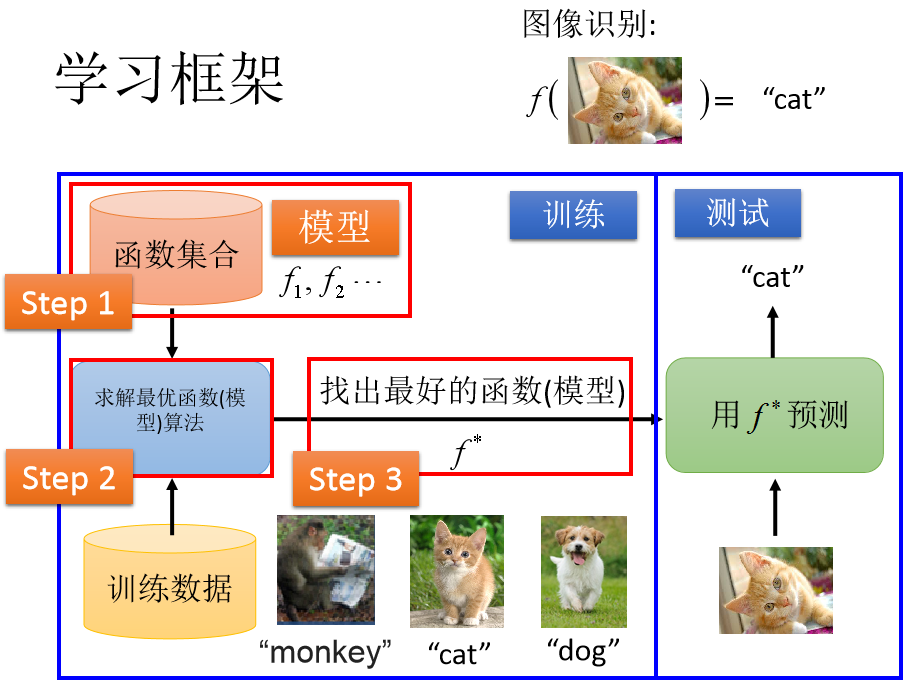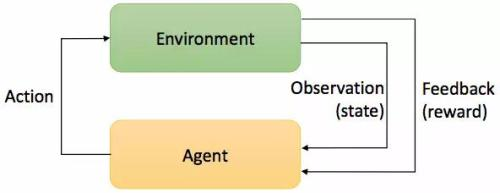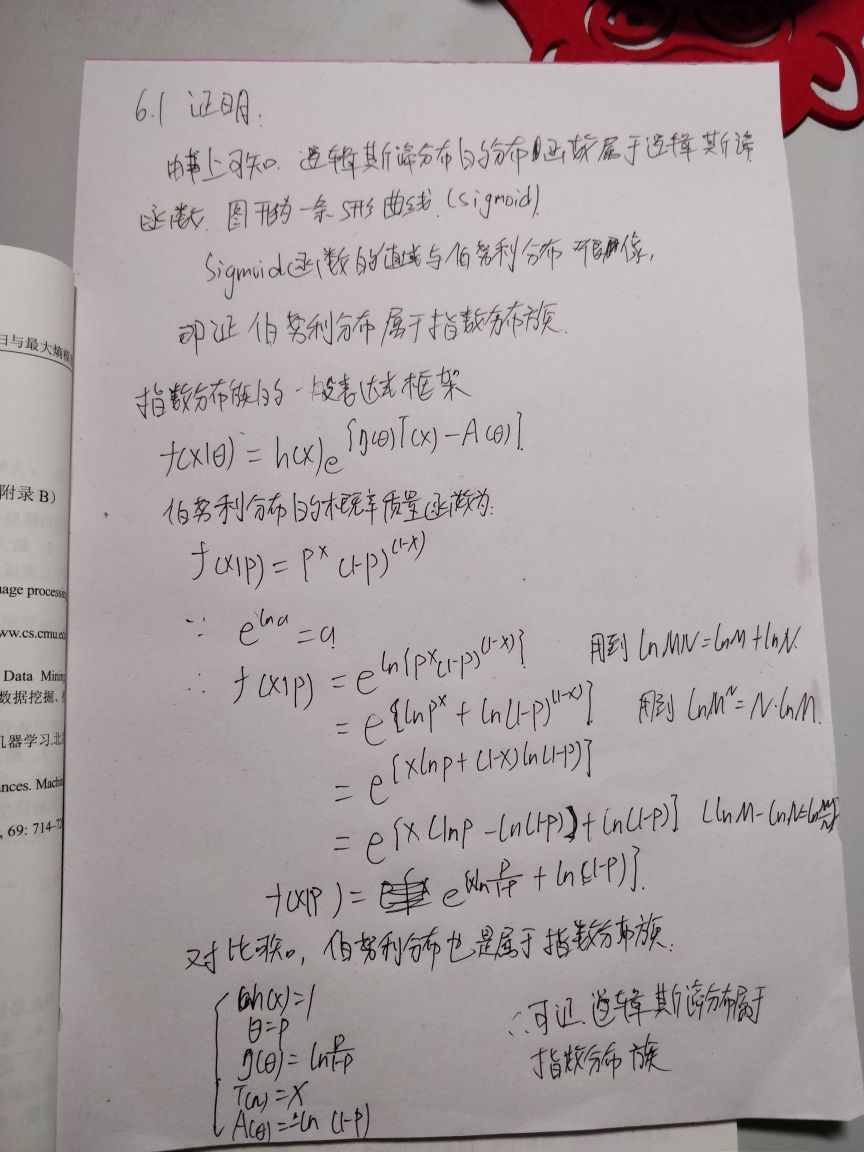2019-07-17 22:30:35 wangwei19871103 阅读数 61

# 目的# 第一章 统计学习及监督学习概论

## 1.1 统计学习

### 统计学习的方法## 1.2 统计学习的分类

### 基本分类

#### 无监督学习

$\displaystyle z=arg \max_{ z} P(z|x)$。一般$z=g(x) $用于降维或者聚类，$P(x|z)$用于概率估计。

#### 强化学习$s$是有限状态state的集合。
$a$是有限动作的action的集合。
$p$是状态转换的概率函数，是条件概率，即在t时刻下状态$s$，采取动作$a$，转换到t+1时刻下的状态$s_{t+1}$的概率，表示为$p(s_{t+1}|s_{t},a_t)$
$r$是奖励函数，即在t时刻下状态$s$，采取动作$a$后获得的奖励，表示为$r(s,a)$
$\gamma$是衰减系数0-1，即认为当前奖励的权重比较大，之后的会衰减。

$v_\pi(s)=E_\pi[r_{t+1}+\gamma r_{t+2}+\gamma^2 r_{t+3}+...|s_t=s]$

$q_\pi(s,a)=E_\pi[r_{t+1}+\gamma r_{t+2}+\gamma^2 r_{t+3}+...|s_t=s,a_t=a]$

### 按技巧分类

#### 贝叶斯学习

$P(\theta|D)=\frac{P(\theta)P(D|\theta)}{P(D)}$
$P(\theta)$是先验概率，就是我们通常知道的概率，$P(D|\theta)$是似然函数，即在参数$\theta$下，样本D来自$P(D|\theta)$的可能性。

$P(x|D)=\int P(x|\theta,D)P(\theta|D)d\theta$
x代表新的样本。

#### 核方法

2017-07-07 13:21:31 l_xyy 阅读数 296

1、什么是监督学习（例如：带有标签的分类问题）。对应着就是无监督学习，半监督学习等。
2、统计学习三要素：模型、策略和算法。
3、在实际应用中，有着精确度等要求，如何在学习过程中验证模型也是很重要的。
4、正则化和交叉验证（懂一点机器学习的人都听过），正则化是一个很有用的东西（深度学习中也是）。
5、泛化能力，就是适应未知数据的能力。
6、生成模型和判别模型（对我来说，判别模型好懂点，生成模型有点抽象，就暂时放放，等看完贝叶斯回头理解一下）。
7、实际问题通常会被转换成分类问题、回归问题等来解决。举例，年龄预测其实应该是一个回归问题，但是在实际应用中通常会转换成分类问题来做。在自然语言处理中，还有标注问题等。

2019-03-26 14:23:53 w_zhao 阅读数 285
10种统计学习方法特点的总结概括

k近邻 多类分类，回归 特征空间，样本点 判别模型

k近邻算法的三要素：距离度量、k值选择、和分类决策规则。

k近邻算法的实现需要考虑如何快速搜索k个最近邻点，kd树是一种便于对k维空间中的数据进行快速检索的数据结构。
kd树是二叉树，对应对k维空间的一个划分，其每个节点对应于k维空间划分中的一个超矩形区域。

EM算法 概率模型参数估计 含隐变量概率模型   极大似然估计，极大后验概率估计 对数似然损失 迭代算法

E步，求期望：

M步，求极大：

2018-08-16 11:59:27 u011500178 阅读数 200

# 李航《统计学习方法》第六章课后习题

## 6.1确认逻辑斯谛分布属于指数分布族2017-08-21 23:00:27 u010978815 阅读数 226
Mark下，周末来写！